﻿ 基于MapReduce的并行异常检测算法
«上一篇文章快速检索 高级检索

 智能系统学报2019, Vol. 14Issue (2): 224-230  DOI: 10.11992/tis.2018090070

### 引用本文QI Xiaogang, HU Qiuqiu, LIU Lifang. Parallel anomaly algorithm based on MapReduce[J]. CAAI Transactions on Intelligent Systems, 2019, 14(2): 224-230. DOI: 10.11992/tis.201809007.### 文章历史

1. 西安电子科技大学 数学与统计学院，陕西 西安 710071;
2. 西安电子科技大学 计算机学院，陕西 西安 710071

Parallel anomaly algorithm based on MapReduce
QI Xiaogang 1, HU Qiuqiu 1, LIU Lifang 2
1. School of Mathematics and Statistics, Xidian University, Xi’an 710071, China;
2. School of Computer Science and Technology, Xidian University, Xi’an 710071, China
Abstract: To improve the accuracy, sensitivity, and efficiency of anomaly detection algorithm in data mining when the amount of data increases, a parallel anomaly detection algorithm (MR-LOF) based on the MapReduce framework and the local outlier factor (LOF) algorithm is proposed in this paper. First, the dataset, stored in the Hadoop distributed file system (HDFS), is logically divided into multiple data blocks. Then, the MapReduce principle is used to process the data in each data block in parallel, so that the k-distance and LOF value of each data point is calculated only in a single block. It greatly improves the efficiency of the algorithm. Simultaneously, the concept of k-distance is redefined. It avoids the situation where the local density is infinite because more than k repeated points exist in the dataset. Finally, the data points whose LOF value is larger than threshold are merged, and the LOF values of combined data are recalculated. This process can effectively improve the accuracy and sensitivity. Experiments with real-world datasets demonstrate the validity, high efficiency, and extendibility of the MR-DLOF algorithm.
Key words: data mining    anomaly detection    local outlier factor    Hadoop    MapReduce    Distributed File System    parallel computing    local density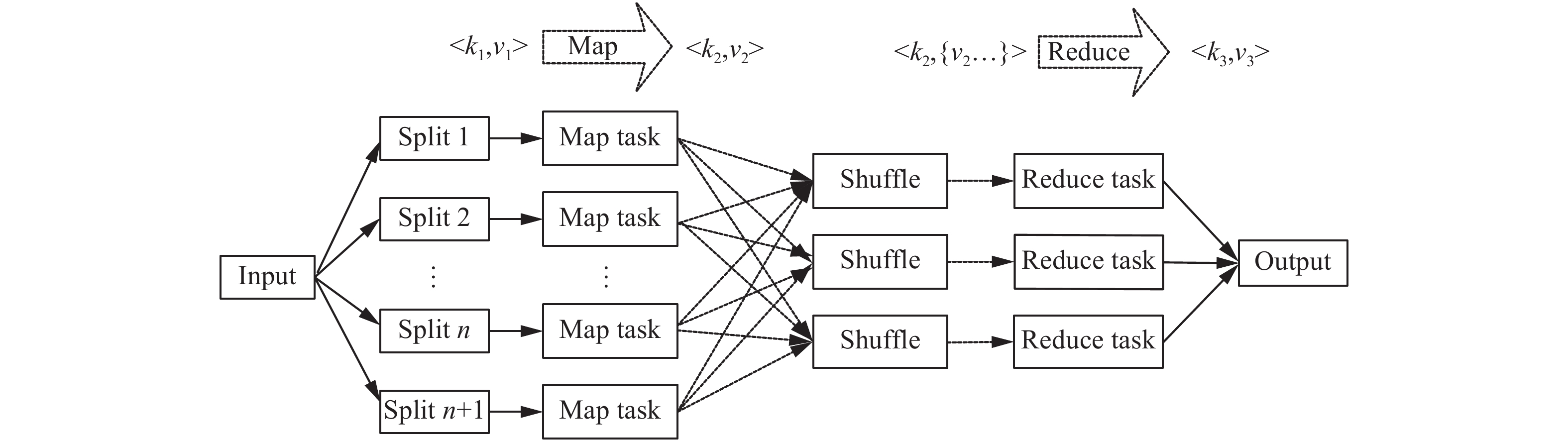Download: 图 1 MapReduce framework处理过程 Fig. 1 MapReduce framework process

Hadoop Distributed File System (HDFS) 采用Master/Slave结构，其作为一个高度容错且易扩展的分布式文件系统，主要包含NameNode、Secondary NameNode和DataNode三类节点图2详细地描述了HDFS结构。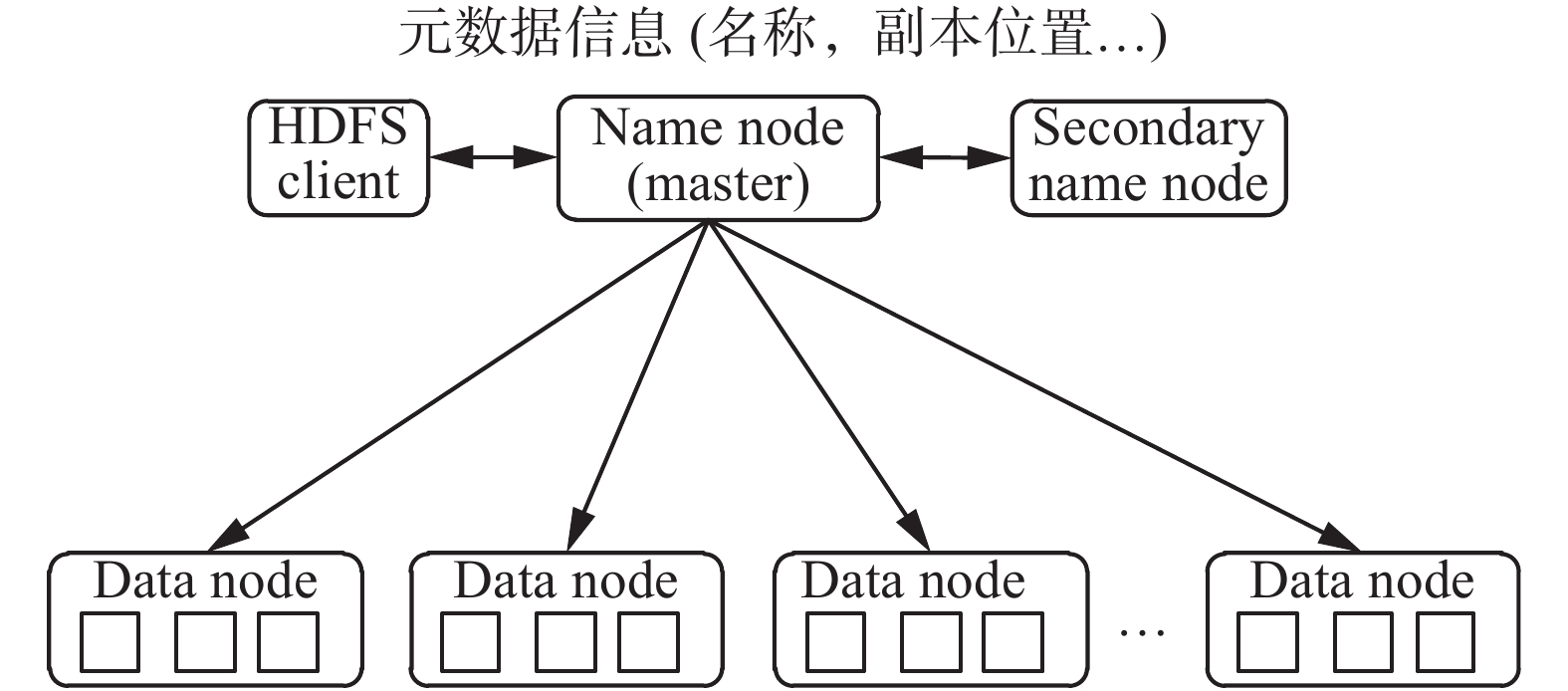Download: 图 2 HDFS结构 Fig. 2 HDFS structure

NameNode为主节点且只有一个，负责接收用户的操作请求，记录每个文件数据块在各个DataNode上的位置和副本信息。Secondary NameNode为可选节点，与NameNode进行通信，定期保存HDFS元数据快照。DataNode为从节点且可以有多个，主要负责存储数据。同时为了保证数据安全，文件会有多个副本(默认为3个)。DataNode定期向NameNode上报心跳，NameNode通过心跳响应来控制DataNode。

2 算法设计 2.1 LOF算法

LOF是基于密度的经典算法，其核心部分是关于数据点密度的刻画。由数据点的k-邻近距离，计算各个数据点的局部可达密度和局部异常因子，根据局部异常因子大小判断数据点的异常程度从而得到异常点。算法的基本概念如下：

1) k-邻近距离 (k-distance): 对于任意正整数 $k$ ，点 $p$ k-邻近距离定义为 $k{\rm{ - }}{\rm distance}{\rm{ }}(p) = d(p,o)$ ，如果满足以下条件：

① 在样本空间中，至少存在 $k$ 个点 $q$ ，使得 $d(p,q) \leqslant d(p,o)$

② 在样本空间中，至多存在 $k - 1$ 个点 $q$ ，使得 $d(p,q) < d(p,o)$

2) k-距离邻域(k-neighbor): 点 $p$ k-距离邻域 ${N_k}(p)$ 即与点 $p$ 的之间距离小于 $k{\rm{ - }}{\rm distance}{\rm{ }}(p)$ 的对象的集合。

3) 可达距离 (reachability distance): 给定参数 $k$ 时，数据点 $p$ 到数据点 $o$ 的可达距离 ${\rm reach}{\rm{ - }}{\rm dis}(p,o)$ 为数据点 $o$ $k{\rm{ - }}{\rm distance}{\rm{ }}(o)$ 和数据点 $p$ 与点 $o$ 之间距离的最大值，即

 ${\rm reach}{\rm{ - }}{{\rm dis}_k}(p,o) = \max \{ k{\rm{ - }}{\rm distance}(o),d(p,o)\}$

4) 局部可达密度 (local reachability density): 数据点 $p$ 的局部可达密度为它与k-距离邻域内数据点的平均可达距离的倒数，即

 $lr{d_k}(p) = \frac{{|{N_k}(p)|}}{{\sum\nolimits_{o \in {N_k}(p)} {{\rm reach}{\rm{ - }}{{\rm dis}_k}(p,o)} }}$ (1)

5) 局部异常因子 (local outlier factor): 数据 $p$ 的局部相对密度(局部异常因子)为点 $p$ 的邻域内点的局部可达密度和数据点 $p$ 的局部可达密度的比值的平均值，即

 ${\rm{LOF}}{_k}(p) = \frac{{\sum\nolimits_{o \in {N_k}(p)} {\displaystyle\frac{{{\rm lrd}(o)}}{{{\rm lrd}(p)}}} }}{{|{N_k}(P)|}}$ (2)

2.2 MR-DLOF算法

LOF算法是基于密度的异常检测算法，计算量大，且在LOF算法中关于局部可达密度的定义存在假设：不存在大于或等于 $k$ 个重复点。当这样的重复点存在的时候，这些点的平均可达距离为零，局部可达密度就变得无穷，从而影响了算法的有效性。

$k$ : number of nearest neighbor

$N$ : data block number

$\theta$ : threshold for LOF

Get data set which $lo{f_i} > \theta$ from 算法 2 add in $XX$

Calculate $lo{f_i} > \theta$ of ${x_j} \in XX$

return Abnormal data and LOF

k-邻近距离(k-distinct-distance): 对于任意正整数 $k$ ，点 $p$ k-邻近距离定义为 $k{\rm{ - }}{\rm distance}{\rm{ }}(p) =$ $d(p,o)$ ，如果满足以下条件：

1) 在样本空间中，至少存在 $k$ 个点 $q$ ，使得 $d(p,q) \leqslant d(p,o)$

2) 在样本空间中，至多存在 $k - 1$ 个点 $q$ ，使得 $0 < d(p,q) < d(p,o)$

$k$ : number of nearest neighbor

$\theta$ : threshold for LOF

$N$ : data block number

Logically divide X into multiple data blocks: ${D_1},{D_2}, \cdots ,{D_N}$ .

FirstMapper

输入　 $D = {d_1},{d_2}, \cdots ,{d_m}$

输出　 $< {\rm key,value} > =$ 　　　　 $< {d_i},\left[ {\left( {{\rm{ }}{o_k},{\rm dis}({d_i},{o_k})} \right),{\rm{ }}k{\rm{ - }}{\rm dis}({d_i})} \right] >$

for each data ${d_i},i = 1,2, \cdots ,m$ do

Calculate ${\rm di{s}_{ij}} = {\rm distance}({d_i},{d_j}),j = 1, \cdots ,m$

Sort ${\rm di{s}_{ij}}$ of ${d_i}$

for each ${\rm di{s}_{ij}}$ of ${d_i}$ do

if ${\rm di{s}_{ij}} \ne 0{\kern 1pt} \& {\kern 1pt} |k{\rm{ - }}{\rm neighbour}| < k$

add ${d_i}$ and ${\rm di{s}_{ij}}$ in k-distinct-neighbor record $\left( {{\rm{ }}{o_k},{\rm dis}({d_i},{o_k})} \right)$

end

Calculate k-distinct-distance record $k{\rm{ - }}{\rm dis}({d_i})$

end

FirstReducer

输入　 $< {\rm key,value} > =$ 　　　　 $< {d_i},\left[ {\left( {{\rm{ }}{o_k},{\rm dis}({d_i},{o_k})} \right),{\rm{ }}k{\rm{ - }}{\rm dis}({d_i})} \right] >$

输出　 $< {\rm key,value} > =$ 　　　　 $< {d_i},\left[ {\left( {{\rm{ }}{o_k},{\rm dis}({d_i},{o_k})} \right),{\rm{ }}k{\rm{ - }}{\rm dis}({d_i})} \right] >$

SecondMapper

输入　 $< {\rm key,value} > =$ 　　　　 $< {d_i},\left[ {\left( {{\rm{ }}{o_k},{\rm dis}({d_i},{o_k})} \right),{\rm{ }}k{\rm{ - }}{\rm dis}({d_i})} \right] >$

输出　 $< {\rm key,value} > =$ 　　　　 $< {d_i},\left( {{\rm{ }}{o_k},{\rm reach}{\rm{ - }}{\rm dis}({d_i},{o_k})} \right) >$

for ${o_k} \in k{\rm{ - }}{\rm distinct}{\rm{ - }}{\rm neighbour}$ do

if $k{\rm{ - }}{\rm dis}({o_k}) < {\rm dis}({d_i},{o_k})$

${\rm reach}{\rm{ - }}{\rm dis}({d_i},{o_k}) = {\rm dis}({d_i},{o_k})$

else ${\rm reach}{\rm{ - }}{\rm dis}({d_i},{o_k}) = k{\rm{ - }}{\rm dis}({d_i},{o_k})$

end

SecondReducer

输入　 $< {\rm key,value} > =$ 　　　　 $< {d_i},\left( {{\rm{ }}{o_k},{\rm reach}{\rm{ - }}{\rm dis}({d_i},{o_k})} \right) >$

输出　 $< {\rm key,value} > {\rm{ = }}$ 　　　　 $< {d_i},{\rm lrd}({d_i}) >$

for value do

${\rm lrd}({d_i}) = k/\sum {{\rm reach}{\rm{ - }}{\rm disk}({d_i},{o_k})}$ , 　　　　　 ${o_k} \in k{\rm{ - }}{\rm distinct}{\rm{ - }}$ neighbour

end

ThirdMapper

输入　 $< {\rm key,value} > = < {d_i},{\rm lrd}({d_i}) >$

输出　 $< {\rm key,value} > =$ 　　　　 $< {d_i}({\rm lof}({d_i}) > \theta ),{\rm lof}({d_i}) >$

for ${o_k} \in k{\rm{ - }}{\rm distinct}{\rm{ - }}{\rm neighbour}$ do

${\rm lof}({d_i}) = (\sum {{\rm lrd}({o_k})/k} )/{\rm lrd}({d_i})$ , 　　　　　 ${o_k} \in k{\rm{ - }}{\rm distinct}{\rm{ - }}$ neighbour

end

if ${\rm lof}({d_i}) > \theta$

output

ThirdReduce

输出　 $< {\rm key,value} > = < {d_i}*,{\rm lof}({d_i}*) >$

for value do

Sort ${\rm lof}({d_i})$ for ${d_i}$ and record ${d_i}*$

end

3 实验与分析表 1 节点信息 Tab.1 Node information

LOF和MR-DLOF算法中采取距离的方法进行计算，由于每个特征属性的度量方法不同，为了避免出现“大数吃小数”的现象，消除属性度量差异对计算结果的影响，需要对数据集进行预处理。本文对除去全为0变量和CSV格式变量后的37个变量进行标准化处理。

3.1 算法的有效性验证表 2 数据检测结果 Tab.2 Data test result

 ${\rm{Accuracy = }}\frac{{{\rm{TP + TN}}}}{{{\rm{TP + TN + FP + FN}}}}$ (3)

 ${\rm{Sensitivit = }}\frac{{{\rm{TN}}}}{{{\rm{TN + FN}}}}$ (4)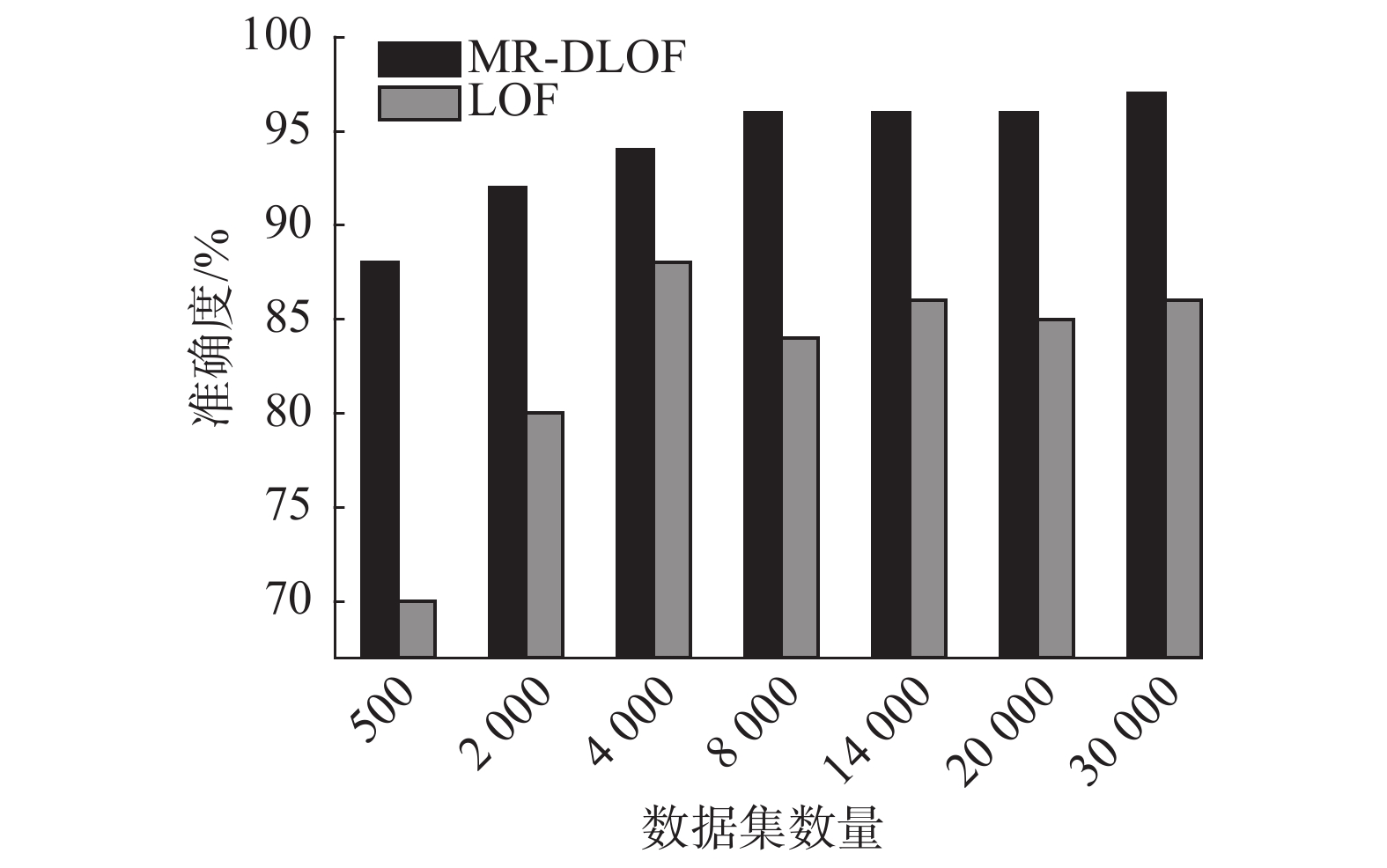Download: 图 3 算法准确度比较 Fig. 3 Accuracy comparison of algorithm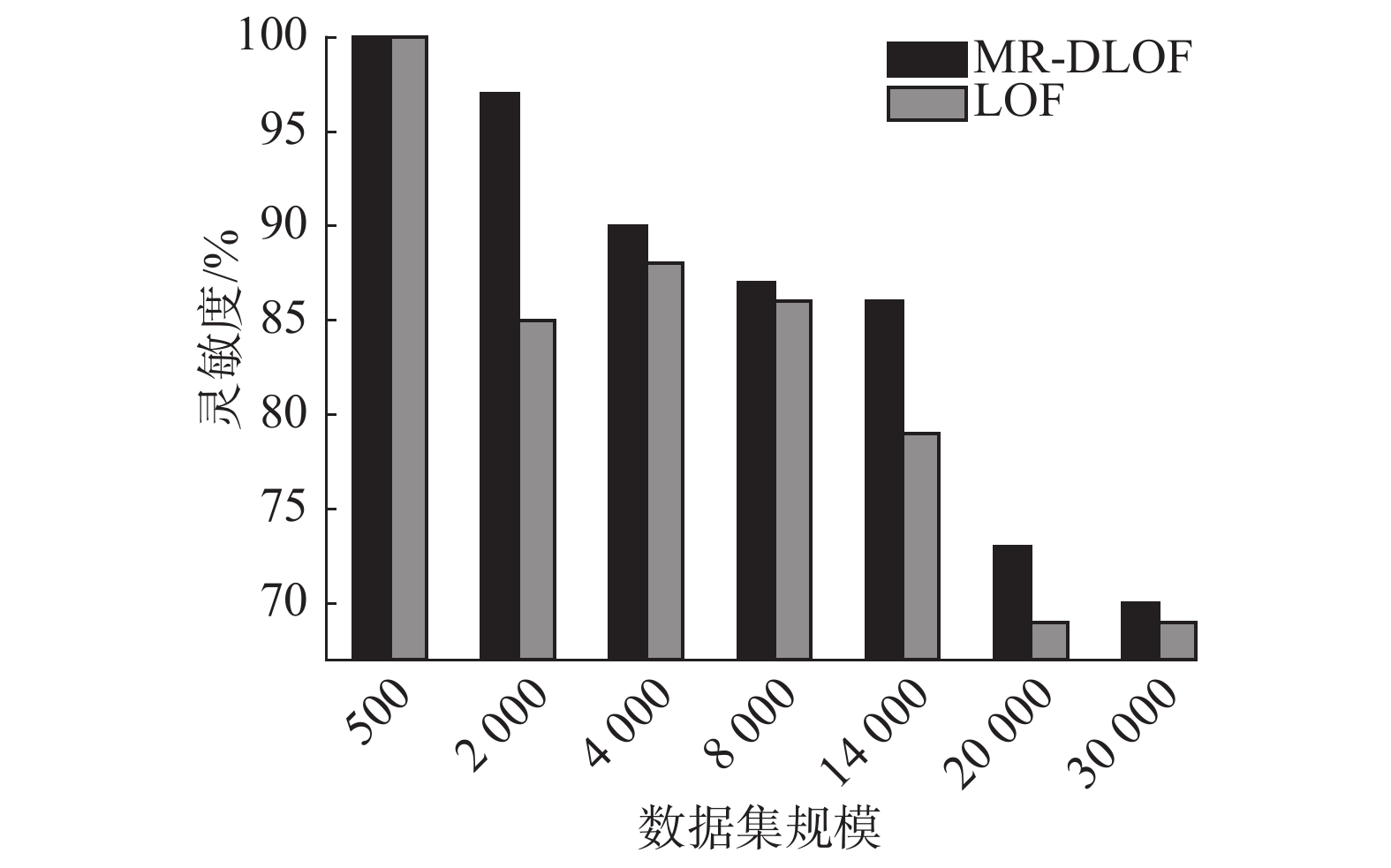Download: 图 4 算法灵敏度比较 Fig. 4 Sensitivity comparison of algorithm
3.2 算法的高效性验证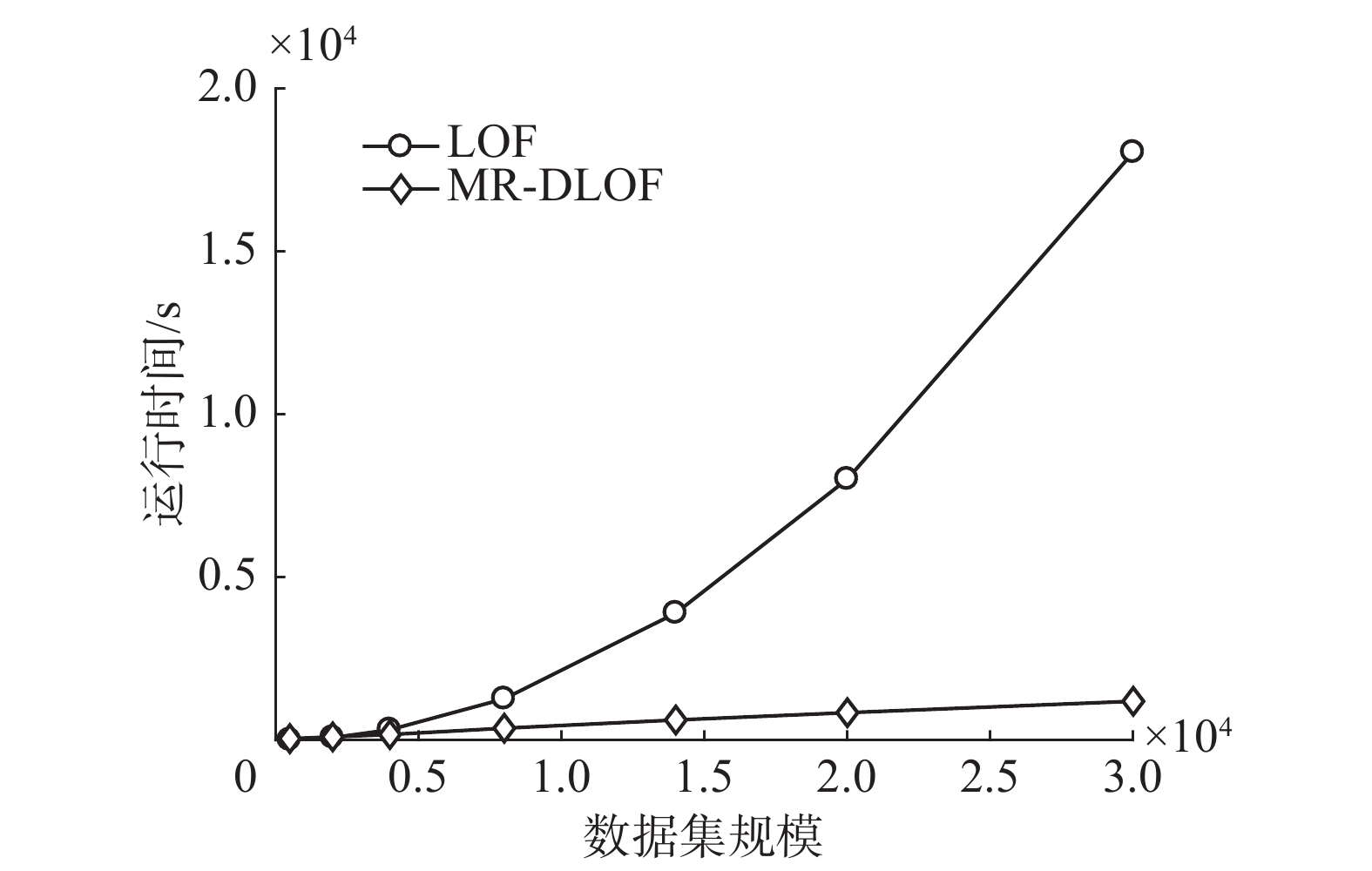Download: 图 5 算法效率比较 Fig. 5 Efficiency comparison of algorithm
3.3 算法的可扩展性验证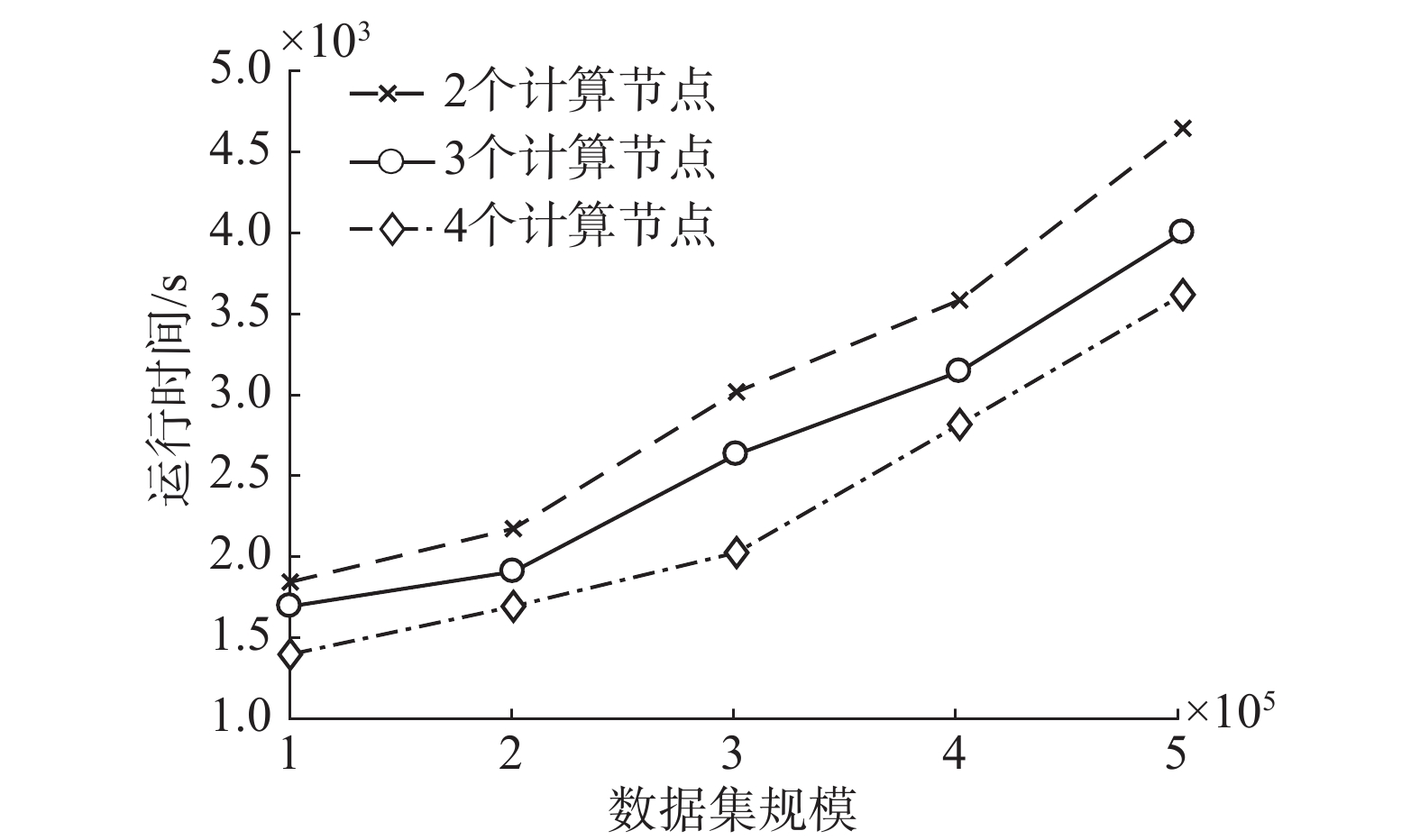Download: 图 6 不同计算节点数量下的执行效率 Fig. 6 Execution efficiency under different calculating node numbers
4 结束语

  HAN Jiawei, KAMBER M. Data mining: concepts and techniques[M]. San Francisco: Morgan Kaufmann Publishers Inc., 2006: 1–18. (0)  AGGARWAL C C. Outlier analysis[M]. New York: Springer, 2013: 75–99. (0)  CHEN Feng, DENG Pan, WAN Jiafu, et al. Data mining for the internet of things: literature review and challenges[J]. International journal of distributed sensor networks, 2015, 2015: 431047. (0)  吴镜锋, 金炜东, 唐鹏. 数据异常的监测技术综述[J]. 计算机科学, 2017, 44(S2): 24-28. WU Jingfeng, JIN Weidong, TANG Peng. Survey on monitoring techniques for data abnormalities[J]. Computer science, 2017, 44(S2): 24-28. (0)  STEINWART I, HUSH D, SCOVEL C. A classification framework for anomaly detection[J]. The journal of machine learning research, 2005, 6(1): 211-232. (0)  邓红莉, 杨韬. 一种基于深度学习的异常检测方法[J]. 信息通信, 2015(3): 3-4. DENG Hongli, YANG Tao. An anomaly detection method based on deep learning[J]. Information and communications, 2015(3): 3-4. DOI:10.3969/j.issn.1673-1131.2015.03.002 (0)  ZHAO Xuanqiang, WANG Guoying, LI Zhixing. Unsupervised network anomaly detection based on abnormality weights and subspace clustering[C]//Proceedings of the 6th International Conference on Information Science and Technology. Dalian, China, 2016: 482–486. (0)  左进, 陈泽茂. 基于改进K均值聚类的异常检测算法[J]. 计算机科学, 2016, 43(8): 258-261. ZUO Jin, CHEN Zemao. Anomaly detection algorithm based on improved K-means clustering[J]. Computer science, 2016, 43(8): 258-261. (0)  邹云峰, 张昕, 宋世渊, 等. 基于局部密度的快速离群点检测算法[J]. 计算机应用, 2017, 37(10): 2932-2937. ZOU Yunfeng, ZHANG Xin, SONG Shiyuan, et al. Fast outlier detection algorithm based on local density[J]. Journal of computer applications, 2017, 37(10): 2932-2937. DOI:10.11772/j.issn.1001-9081.2017.10.2932 (0)  BREUNIG M M, KRIEGEL H P, NG R T, et al. LOF: identifying density-based local outliers[C]//Proceedings of 2000 ACM SIGMOD International Conference on Management of Data. Dallas, Texas, USA, 2000: 93–104. (0)  BHATT V, SHARMA K G, RAM A. An enhanced approach for LOF in data mining[C]//Proceedings of 2013 International Conference on Green High Performance Computing. Nagercoil, India, 2013: 1–3. (0)  MIAO Dandan, QIN Xiaowei, WANG Weidong. Anomalous cell detection with kernel density-based local outlier factor[J]. China communications, 2015, 12(9): 64-75. DOI:10.1109/CC.2015.7275260 (0)  TANG Bo, HE Haibo. A local density-based approach for outlier detection[J]. Neurocomputing, 2017, 241: 171-180. DOI:10.1016/j.neucom.2017.02.039 (0)  DEAN J, GHEMAWAT S. MapReduce: simplified data processing on large clusters[J]. Communications of the ACM, 2008, 51(1): 107-113. DOI:10.1145/1327452 (0)  GHEMAWAT S, GOBIOFF H, LEUNG S T. The google file system[C]//Proceedings of the 19th ACM Symposium on Operating Systems Principles. Bolton Landing, NY, USA, 2003: 29–43. (0)  CHANG F, DEAN J, GHEMAWAT S, et al. Bigtable: a distributed storage system for structured data[C]//Proceedings of the 7th USENIX Symposium on Operating Systems Design and Implementation. Seattle, WA, 2006: 15. (0)  MU Yashuang, LIU Xiaodong, YANG Zhihao, et al. A parallel C4.5 decision tree algorithm based on MapReduce[J]. Concurrency and computation practice and experience, 2017, 29(8): e4015. DOI:10.1002/cpe.4015 (0)  侯泳旭, 段磊, 秦江龙, 等. 基于Isolation Forest的并行化异常探测设计[J]. 计算机工程与科学, 2017, 39(2): 236-244. HOU Yongxu, DUAN Lei, QIN Jianglong, et al. Parallel anomaly detection based on isolation forest[J]. Computer engineering & science, 2017, 39(2): 236-244. DOI:10.3969/j.issn.1007-130X.2017.02.003 (0)  叶海琴, 孟彩霞, 王意锋, 等. 一种基于MapReduce的频繁模式挖掘算法[J]. 南京理工大学学报, 2018, 42(1): 62-67. YE Haiqin, MENG Caixia, WANG Yifeng, et al. Frequent pattern mining algorithm based on MapReduce[J]. Journal of Nanjing University of Science and Technology, 2018, 42(1): 62-67. (0)  刘义, 景宁, 陈荦, 等. MapReduce框架下基于R-树的k-近邻连接算法[J]. 软件学报, 2013, 24(8): 1836-1851. LIU Yi, JING Ning, CHEN Luo, et al. Algorithm for processing k-nearest join based on R-tree in MapReduce[J]. Journal of software, 2013, 24(8): 1836-1851. (0)  WHITE T. Hadoop: the definitive guide[M]. 4th ed. Gravenstein Highway North: O’reilly Media Inc., 2015: 1–4. (0)  http://archive.ics.uci.edu/ml/machine-learning-databases/kddcup99-mld/. (0)